Back

# TileDB 101: GeometriesGeospatial Data Scientist

This article covers the basics of how to use TileDB arrays with geospatial vector data. I will show how to ingest the geometries from a shapefile into a TileDB array and how to then read the data from this array. I will also show how to use the polygons from the geometry array to slice and read data from a point cloud (also ingested into a TileDB array). Before proceeding with these tutorials, I recommend you read the blog Why TileDB for Geometries. In this introductory tutorial I will focus on a geospatial application, noting however that TileDB’s geometries feature is applicable to other use cases as well (such as spatial transcriptomics — we’ll cover other use cases in future tutorials).

Geospatial vector data contains features such as points, lines and polygons represented by geometry. The geometry consists of connected vertices that describe a position in space with `X` and `Y`. The TileDB geometry functionality is based on the OGR Simple Features Model. The geometries are efficiently stored as Well Known Binary (WKB), which is a scheme for writing a Simple Features geometry into an array of bytes. This is implemented in the vector data model of GDAL and in other spatial databases such as MariaDB, Oracle and PostGIS. From GDAL 3.7.1 it can also be used with TileDB arrays where the geometry is stored in a binary blob attribute (`wkb_geometry`) containing WKB geometries.

To query at scale it is also necessary to have a spatial index on your data. Without a spatial index every object in your database will be queried to apply the spatial operator, which is known as a full scan. TileDB natively builds an R-Tree on your data, which is a multi-dimensional index that enables you to quickly and efficiently access only the spatial region of the data you are querying.

# Setup

This tutorial uses both GDAL, PDAL and various other geospatial Python packages. Installing these together with either `conda` or `mamba` is difficult because of the many dependencies between the various packages. Therefore, I created a Dockerfile that installs the packages from source. With this you can run this notebook locally.

Alternatively, you can run the tutorial notebook in a JupyterLab environment on TileDB Cloud (sign up and you will receive free credits), which comes with all the necessary packages pre-installed. If you’d like to make modifications to the notebook, you can copy the notebook to your account with the`Copy`button, then launch a notebook server on TileDB Cloud by clicking the `Launch` button from the preview page on your copy of the notebook.

# Data ingestion

First, we will import all the required libraries:

``````import os
import shutil
import fiona
import shapely
from shapely import wkb
import geopandas as gpd
import matplotlib.pyplot as plt
from pybabylonjs import Show as show
import pdal
import tiledb
``````

Enable TileDB as a supported driver with the `fiona` library, which is a pythonic wrapper around many GDAL vector (OGR) drivers:

``````fiona.drvsupport.supported_drivers["TileDB"] = "rw"
``````

Data of the building outlines in New Zealand is used in this notebook (CC by 4.0 license). I signed up for an account, downloaded the shapefile, and stored the file as a TileDB Cloud array (yep, TileDB can also store files as arrays, which you can download into their original file format by simply clicking on the “download” button).

For this notebook I also created a smaller file that you can download and unzip to your local directory as below with a free TileDB Cloud account. Or go to the public array of this file and click on the download button and save the unzipped files to the `geometry101` local directory.

``````geom_dir = os.path.expanduser("~/geometry101/")
os.makedirs(geom_dir)

tiledb.cloud.file.export_file(
output_uri=os.path.expanduser("~/geometry101/buildings.zip"),
)

shutil.unpack_archive(os.path.expanduser("~/geometry101/buildings.zip"),
os.path.expanduser("~/geometry101/"))
``````

You can now ingest the data into a sparse TileDB array with ogr2ogr, which is a command line tool that converts simple features data between file formats:

``````shape_file = os.path.expanduser("~/geometry101/buildings/buildings.shp")
buildings_array = "arrays/NZ-buildings"

!ogr2ogr -f TileDB \$buildings_array \$shape_file
``````

Let's find out what is in the new array with the geometries. Use the TileDB API to print the schema of the array:

``````with tiledb.Array(buildings_array) as A:
print(A.schema)
``````

Which gives:

``````ArraySchema(
domain=Domain(*[
Dim(name='_X', domain=(-40075016.68557849, 40075016.68557849), tile=8015003.337115698, dtype='float64'),
Dim(name='_Y', domain=(-40075016.68557849, 40075016.68557849), tile=8015003.337115698, dtype='float64'),
]),
attrs=[
Attr(name='FID', dtype='int64', var=False, nullable=False),
Attr(name='wkb_geometry', dtype='blob', var=True, nullable=False),
Attr(name='building_i', dtype='int32', var=False, nullable=True),
Attr(name='name', dtype='<U0', var=True, nullable=True),
Attr(name='use', dtype='<U0', var=True, nullable=True),
Attr(name='suburb_loc', dtype='<U0', var=True, nullable=True),
Attr(name='town_city', dtype='<U0', var=True, nullable=True),
Attr(name='territoria', dtype='<U0', var=True, nullable=True),
Attr(name='capture_me', dtype='<U0', var=True, nullable=True),
Attr(name='capture_so', dtype='<U0', var=True, nullable=True),
Attr(name='capture__1', dtype='int32', var=False, nullable=True),
Attr(name='capture__2', dtype='<U0', var=True, nullable=True),
Attr(name='capture__3', dtype='datetime64[D]', var=False, nullable=True),
Attr(name='capture__4', dtype='datetime64[D]', var=False, nullable=True),
Attr(name='last_modif', dtype='datetime64[D]', var=False, nullable=True),
],
cell_order='row-major',
tile_order='row-major',
capacity=10000,
sparse=True,
allows_duplicates=True,
)
``````

From the above schema you can see that the array is sparse (`sparse=True`) and that the domain contains `_X` and `_Y`, which are the centers of each geometry used in the fast spatial queries. The values used to pad the query extents are stored as metadata in the TileDB array with keys `PAD_X` and `PAD_Y`.

The GDAL application layer also allows the `_X` and `_Y` dimensions to be configured to different names. `Z` is supported as well and this is known as `2.5D`as the geometries are calculated in the X/Y plane. The geometries are in the `wkb_geometry` attribute and this has a blob datatype. To read this data it can be converted with other tools such as `ogrinfo` or `geopandas`, which I will show below.

# Spatial queries

## Spatial query for one building

With `ogrinfo` I will select and read the features inside an area of interest or bounding box, which is defined with `-spat <xmin> <ymin> <xmax> <ymax>`. Adding `-al` will show all layers. Because of the use of a spatial index and R-tree in TileDB sparse arrays this is very fast. Without an indexed dimension query, a full scan over all geometries would be needed, which would take a lot longer.

``````!ogrinfo -al \$buildings_array -spat 1749923 5946204 1749924 5946205
``````

Which gives the below (note that some lines are removed for clarity):

``````INFO: Open of `/home/jovyan/geometry101/NZ-buildings'
using driver `TileDB' successful.

Layer name: NZ-buildings
Geometry: Polygon
Feature Count: 1
Extent: (1580475.945188, 5174979.602674) - (1750351.532268, 5947023.813595)
Layer SRS WKT:
PROJCRS["NZGD2000 / New Zealand Transverse Mercator 2000",
BASEGEOGCRS["NZGD2000",
...
capture__4 (Date) = 2017/05/06
last_modif (Date) = 2019/03/26
POLYGON ((1749923.14111584 5946204.20193389,1749923.19014299 5946186.44729398,1749907.98990394 5946190.89433034,1749908.03921575 5946198.38781489,1749902.48726419 5946198.32433004,1749902.79002773 5946203.44223618,1749907.9745329 5946203.44609882,1749907.97397819 5946204.19063372,1749923.14111584 5946204.20193389))
``````

I can use other geospatial libraries to read data for the same area of interest, because the GDAL OGR driver is integrated with them. When I enabled TileDB as a supported driver with `fiona` above, it also made it available in `geopandas`. Below I load the same building from the TileDB array directly in a `geopandas` dataframe with the same bounding box (`bbox`) and then plot it:

``````%%time
gdf = gpd.read_file(buildings_array, bbox=(1749923, 5946204, 1749924, 5946205))

CPU times: user 44.2 ms, sys: 269 ms, total: 313 ms
Wall time: 216 ms
``````

To increase the speed of spatial queries with `geopandas` I can add the pyogrio engine and also enable the use of `arrow`. Traditional geospatial data access with for instance `fiona` uses a row layout. The `pyogrio` interface uses the Arrow Batch Table interface in GDAL OGR to load the data in a column layout in batches. This will make the reads of geometries and their attributes very fast, as well as the calculations performed on the attribute values. Below you will only see a small difference, but with very large queries this increase in speed is much larger.

``````%%time
gdf = gpd.read_file(buildings_array, engine="pyogrio", use_arrow=True, bbox=(1749923, 5946204, 1749924, 5946205))
``````
``````CPU times: user 98.2 ms, sys: 57.6 ms, total: 156 ms
Wall time: 80.7 ms
``````

The geopandas dataframe containing a single building can be plotted:

``````gdf.plot()
``````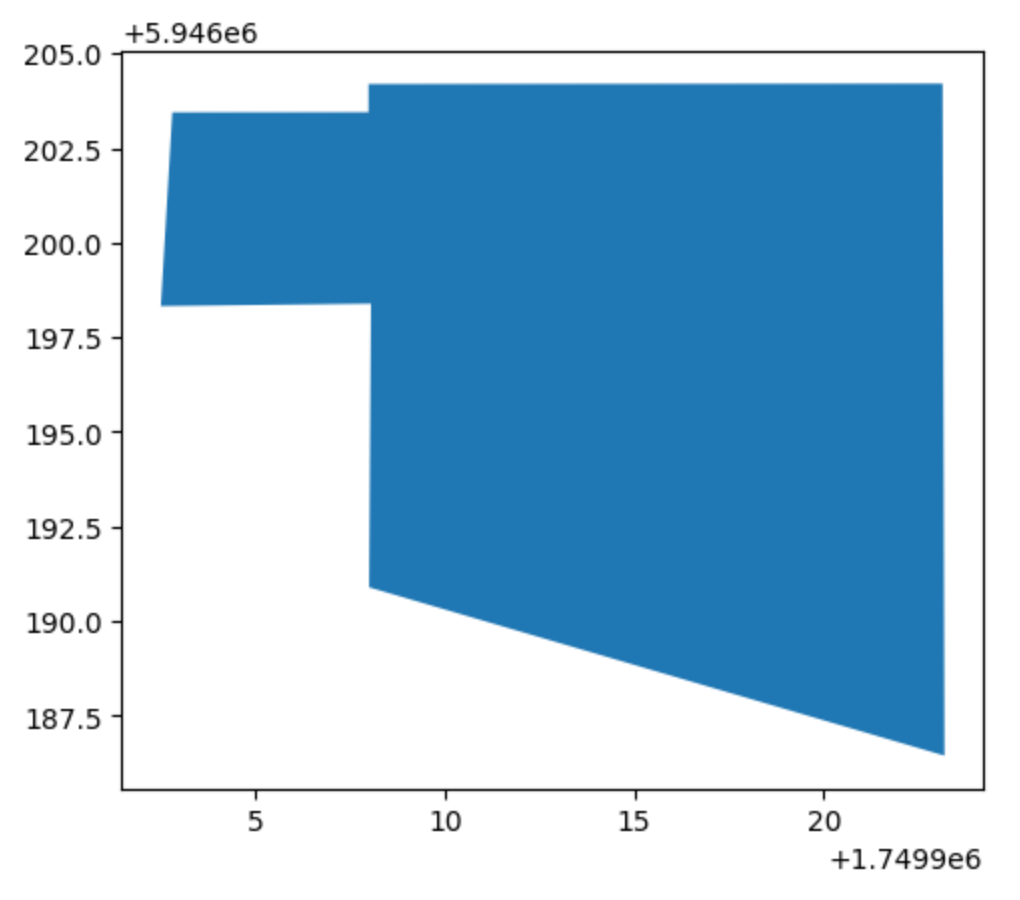## Spatial query for a larger area

Let's increase the area of interest and read more polygons. I will now add `-so` to get a summary only, and note that below some lines are removed for clarity:

``````!ogrinfo \$buildings_array -al -so -spat 1700000 5500000 1800000 560000
``````
``````INFO: Open of `/home/jovyan/geometry101/NZ-buildings'
using driver `TileDB' successful.

Layer name: NZ-buildings
Geometry: Polygon
Feature Count: 4639
Extent: (1580475.945188, 5174979.602674) - (1750351.532268, 5947023.813595)
Layer SRS WKT:
PROJCRS["NZGD2000 / New Zealand Transverse Mercator 2000",
...
capture__2: String (0.0)
capture__3: Date (0.0)
capture__4: Date (0.0)
last_modif: Date (0.0)
``````

And I will perform the same query with geopandas that is now very fast because of the addition of the `pyogrio` engine. When I ran the below without, it was over 5 times slower!

``````%%time
gdf1 = gpd.read_file(buildings_array, engine="pyogrio", use_arrow=True, bbox=(1700000, 5500000, 1800000, 560000))
gdf1
``````
``````CPU times: user 221 ms, sys: 198 ms, total: 419 ms
Wall time: 256 ms
``````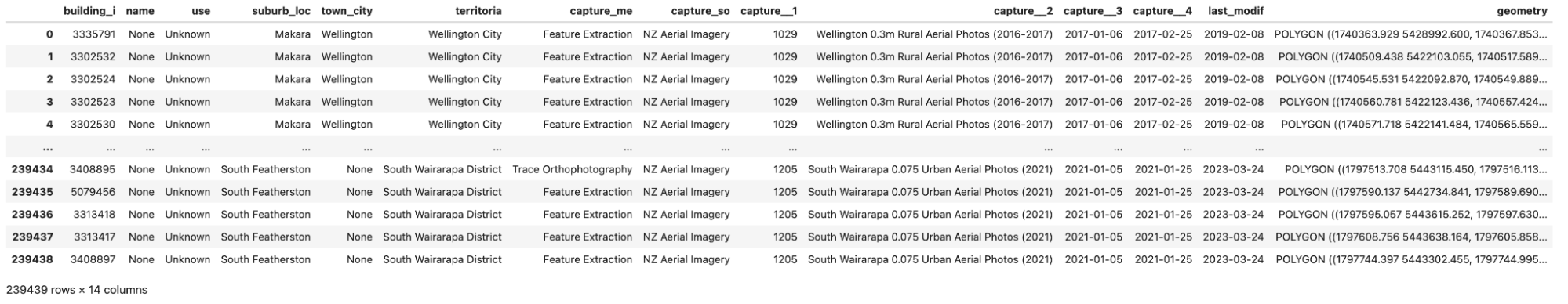## More complex spatial queries

So far I have queried the data with a rectangular area of interest or bounding box, but other shapes can be used as well. Let's do a typical geospatial operation with a query for an area with a defined radius around a point. Note that the `pyogrio` engine currently only supports `bbox` queries and therefore needs a post-filter to query for geometries that intersect the circle.

I create a circle with a 100 meter buffer around a point:

``````circle = shapely.buffer(shapely.Point(1749923, 5946204), 100)
circle
``````And then query and plot the features that intersect with the circle:

``````gdf2 = gpd.read_file(buildings_array, engine="pyogrio", use_arrow=True, bbox=circle.bounds)

for r in range(len(gdf2)):
if gdf2.geometry[r].intersects(circle):
plt.plot(*gdf2.geometry[r].exterior.xy)

plt.plot(*circle.exterior.xy)
plt.title("Spatial Query")
plt.xlabel("X")
plt.ylabel("Y")

plt.show()
``````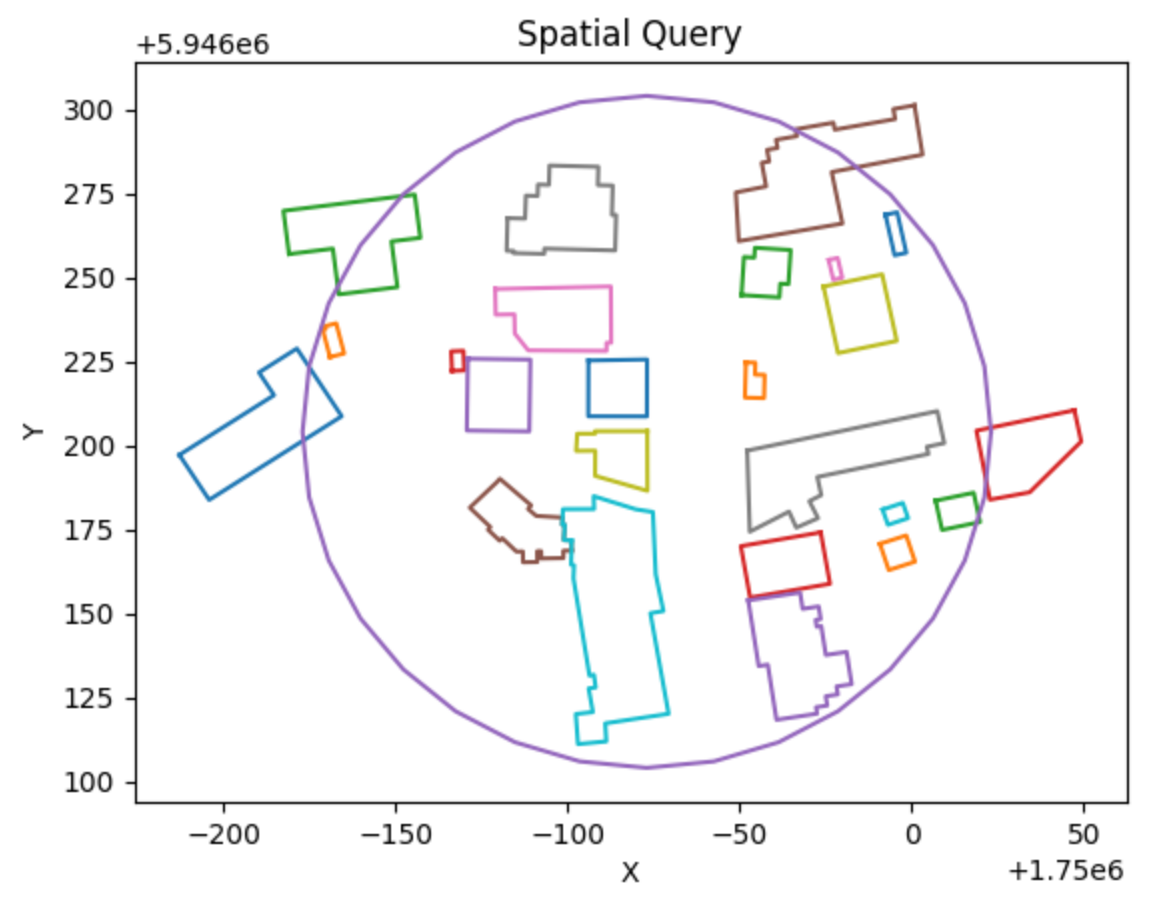Have a look at the geometries above that intersect with the circle. Most of them have a center that is outside the circle. From the below query you can see why this is correct and the same as a query with the circle directly. Here I do not use `pyogrio`, which means I can do the query directly with the circle as a `mask`. In this case the padding values from the metadata are used to increase the size of the mask. These padding values are calculated from all features and are the maximum values of the length in the `X` and `Y` direction. This will make sure that all features intersecting with the circle are included.

``````gdf3 = gpd.read_file(buildings_array, use_arrow=True, mask = circle)

[fig, ax] = plt.subplots(1, figsize=(10, 6))
plt.plot(*circle.exterior.xy)
gdf3.plot(ax=ax,color='#D81B60')
ax.axis('off');
``````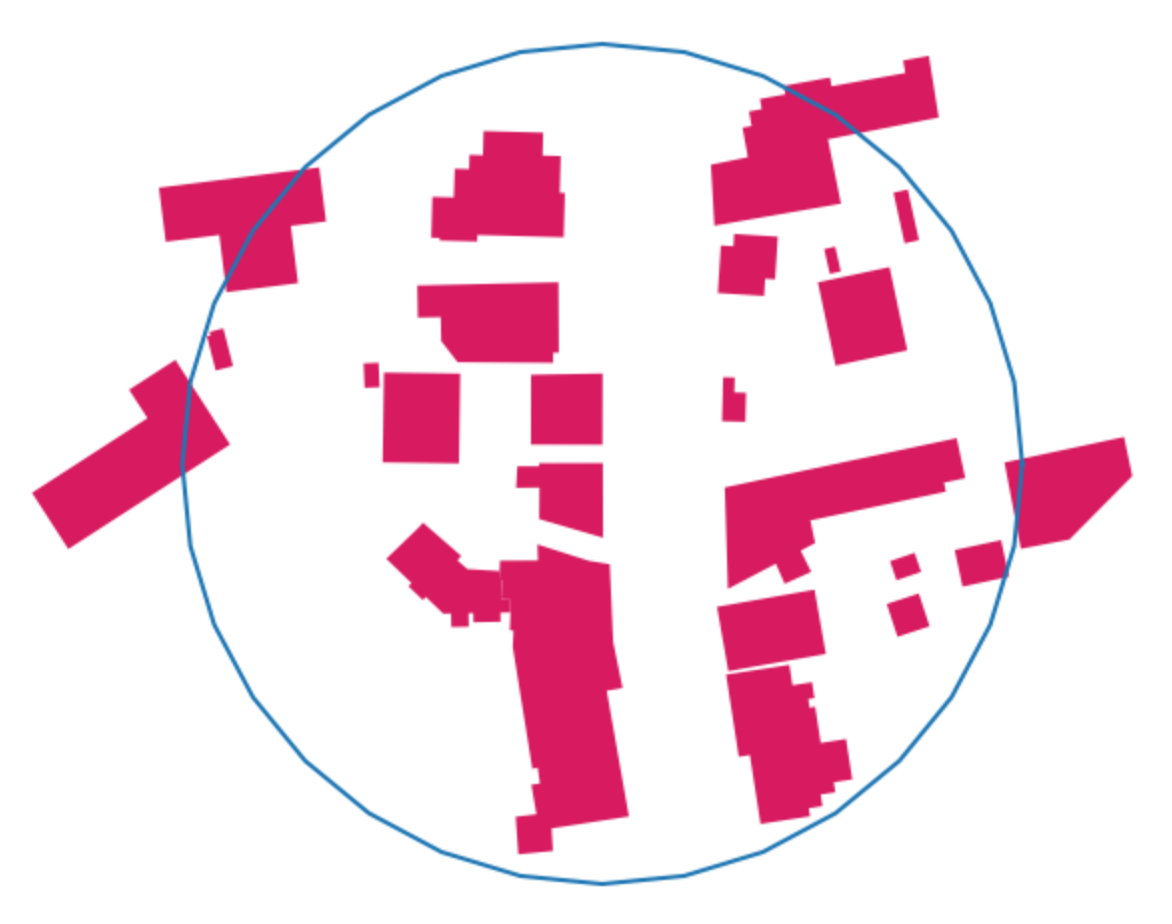With our MariaDB integration, I can perform the same query by writing the buffer and spatial intersection in SQL.

``````SELECT name, GeometryFromWkb(wkb_geometry) as geom
FROM `/path/to/arrays/NZ-buildings`
WHERE ST_Intersects(
GeometryFromWkb(wkb_geometry),
ST_Buffer(GeometryFromText('POINT(1749923 5946204)'), 100.0)
);
``````

# Point cloud spatial queries

Now something different!

I will show how to create a point cloud with PDAL and then use the geometry features from the buildings array to read the points for the area of a building. The point cloud data is from the same data source and the same coordinate reference system as the buildings data.

Through the OpenTopography portal I selected a bounding box (`xmin=1580700`, `ymin=5175200`, `xmax=1580900`, and `ymax=5175500`) and then downloaded the point cloud data as a LAZ file. Similar to the buildings shape file, I stored the file as a TileDB Cloud array.

Copy the file from the TileDB Cloud array:

``````tiledb.cloud.file.export_file(
uri="tiledb://TileDB-Inc/218121b2-c9ab-48c0-8ea4-5fceed74da75",
output_uri=os.path.expanduser("~/geometry101/points.laz"),
)
``````

The point cloud can now be ingested into a TileDB sparse array in the same way as is described in the TileDB 101: Point Clouds blog.

``````laz_file = os.path.expanduser("~/geometry101/points.laz")
points_array = os.path.expanduser("~/geometry101/NZ-points")

pipeline |= pdal.Writer.tiledb(array_name=points_array, x_tile_size=1000, y_tile_size=1000, z_tile_size=100, chunk_size=1_500_000)
pipeline.execute()
``````

Let's first review the point cloud schema, which is the standard point cloud layout:

``````with tiledb.open(points_array) as arr:
print(arr.schema)
``````

Which gives:

``````ArraySchema(
domain=Domain(*[
Dim(name='X', domain=(-1.7976931348623157e+308, 1.7976931348623157e+308), tile=1000.0, dtype='float64', filters=FilterList([FloatScaleFilter(factor=0.01,offset=0.0,bytewidth=4), DeltaFilter(), BitShuffleFilter(), ZstdFilter(level=7), ])),
Dim(name='Y', domain=(-1.7976931348623157e+308, 1.7976931348623157e+308), tile=1000.0, dtype='float64', filters=FilterList([FloatScaleFilter(factor=0.01,offset=0.0,bytewidth=4), DeltaFilter(), BitShuffleFilter(), ZstdFilter(level=7), ])),
Dim(name='Z', domain=(-1.7976931348623157e+308, 1.7976931348623157e+308), tile=100.0, dtype='float64', filters=FilterList([FloatScaleFilter(factor=0.01,offset=0.0,bytewidth=4), DeltaFilter(), BitShuffleFilter(), ZstdFilter(level=7), ])),
]),
attrs=[
Attr(name='Intensity', dtype='uint16', var=False, nullable=False, filters=FilterList([DeltaFilter(), ZstdFilter(level=5), ])),
Attr(name='ReturnNumber', dtype='uint8', var=False, nullable=False, filters=FilterList([ZstdFilter(level=5), ])),
Attr(name='NumberOfReturns', dtype='uint8', var=False, nullable=False, filters=FilterList([ZstdFilter(level=5), ])),
Attr(name='ScanDirectionFlag', dtype='uint8', var=False, nullable=False, filters=FilterList([ZstdFilter(level=5), ])),
Attr(name='EdgeOfFlightLine', dtype='uint8', var=False, nullable=False, filters=FilterList([ZstdFilter(level=5), ])),
Attr(name='Classification', dtype='uint8', var=False, nullable=False, filters=FilterList([ZstdFilter(level=5), ])),
Attr(name='ScanAngleRank', dtype='float32', var=False, nullable=False, filters=FilterList([ZstdFilter(level=5), ])),
Attr(name='UserData', dtype='uint8', var=False, nullable=False, filters=FilterList([ZstdFilter(level=5), ])),
Attr(name='PointSourceId', dtype='uint16', var=False, nullable=False, filters=FilterList([ZstdFilter(level=5), ])),
Attr(name='GpsTime', dtype='float64', var=False, nullable=False, filters=FilterList([DeltaFilter(), BitWidthReductionFilter(window=256), ZstdFilter(level=7), ])),
Attr(name='ScanChannel', dtype='uint8', var=False, nullable=False),
Attr(name='ClassFlags', dtype='uint8', var=False, nullable=False),
],
cell_order='row-major',
tile_order='row-major',
capacity=100000,
sparse=True,
allows_duplicates=True,
)
``````

I can also review the point cloud with the GDAL OGR tools:

``````!ogrinfo -al -so \$points_array
``````

Which gives:

``````INFO: Open of `/home/jovyan/geometry101/NZ-points'
using driver `TileDB' successful.

Layer name: NZ-points
Geometry: 3D Point
Feature Count: 1239441
Extent: (1580700.000000, 5175200.001000) - (1580899.999000, 5175499.999000)
Layer SRS WKT:
(unknown)
Intensity: Integer (0.0) NOT NULL
...
``````

I will now go back to the buildings array to read the geometry of a single building:

``````with tiledb.open(buildings_array) as src:
q = src.query(cond="attr('use') != 'Unknown'", attrs=["name", "use", "wkb_geometry"], use_arrow=False)
df = q.df[:]

wkb_geom = df.query('name.str.contains("Our Lady Star of the Sea School")', engine="python").iloc.wkb_geometry.tobytes()

geom
``````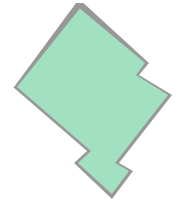I can now use this geometry to load the corresponding points from the point cloud:

``````gpdf = gpd.read_file(points_array, engine="pyogrio", use_arrow=True, bbox=geom.bounds)
gpdf["Red"] = 0.0
gpdf["Green"] = 0.0
gpdf["Blue"] = 0.0
msk = gpdf.within(geom)
school_points = gpdf.loc[msk]
school_points.plot()
``````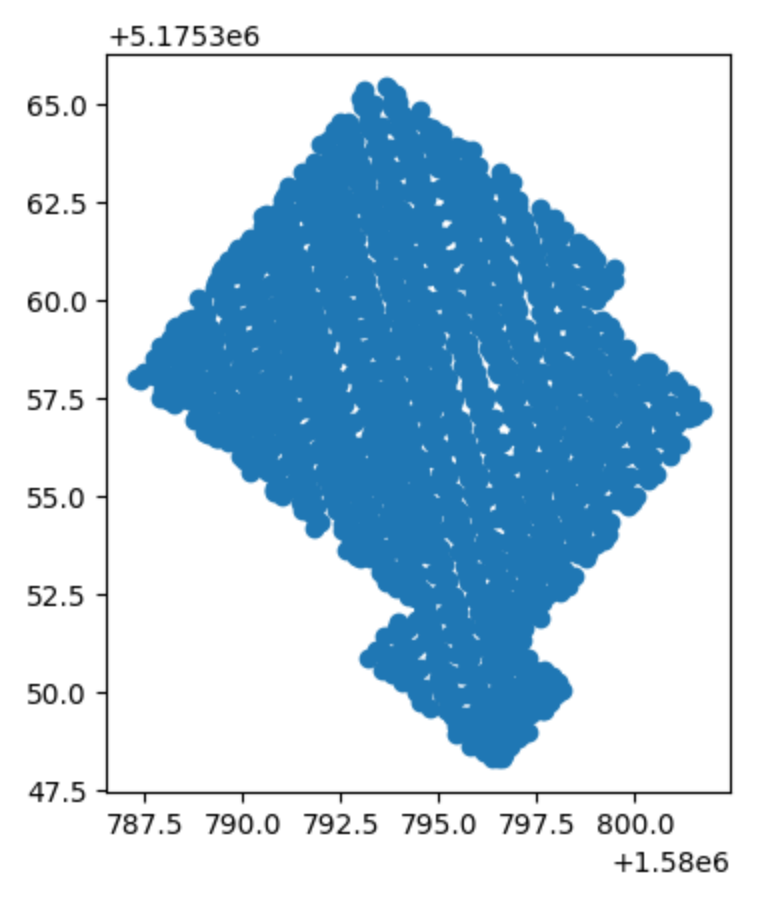As I am only interested in the building, let's check for any misclassifications from the extracted points. The classification codes are defined here and class `6` is a building. A quick way to inspect this is by visualizing the building and the misclassified points using the TileDB integration with BabylonJS.

Before visualizing the points I color the building points red and the vegetation points green. I load the data into a dictionary and then use `show.point_cloud()` to create the interactive visualization. In the viewer, I will be able to use my mouse to:

• Zoom in and out with the scroll wheel
• Rotate by dragging the mouse with the left button down
``````school_points.loc[school_points["Classification"] == 6, "Red"] = 0.5
school_points.loc[school_points["Classification"] != 6, "Green"] = 1.0

data = {
"X": school_points.geometry.x,
"Y": school_points.geometry.y,
"Z": school_points.geometry.z,
"Red": school_points["Red"],
"Green": school_points["Green"],
"Blue": school_points["Blue"]
}

show.point_cloud(source="dict",
data=data,
point_size=8,
rgb_max=1.0,
width = 1000,
height = 600)
``````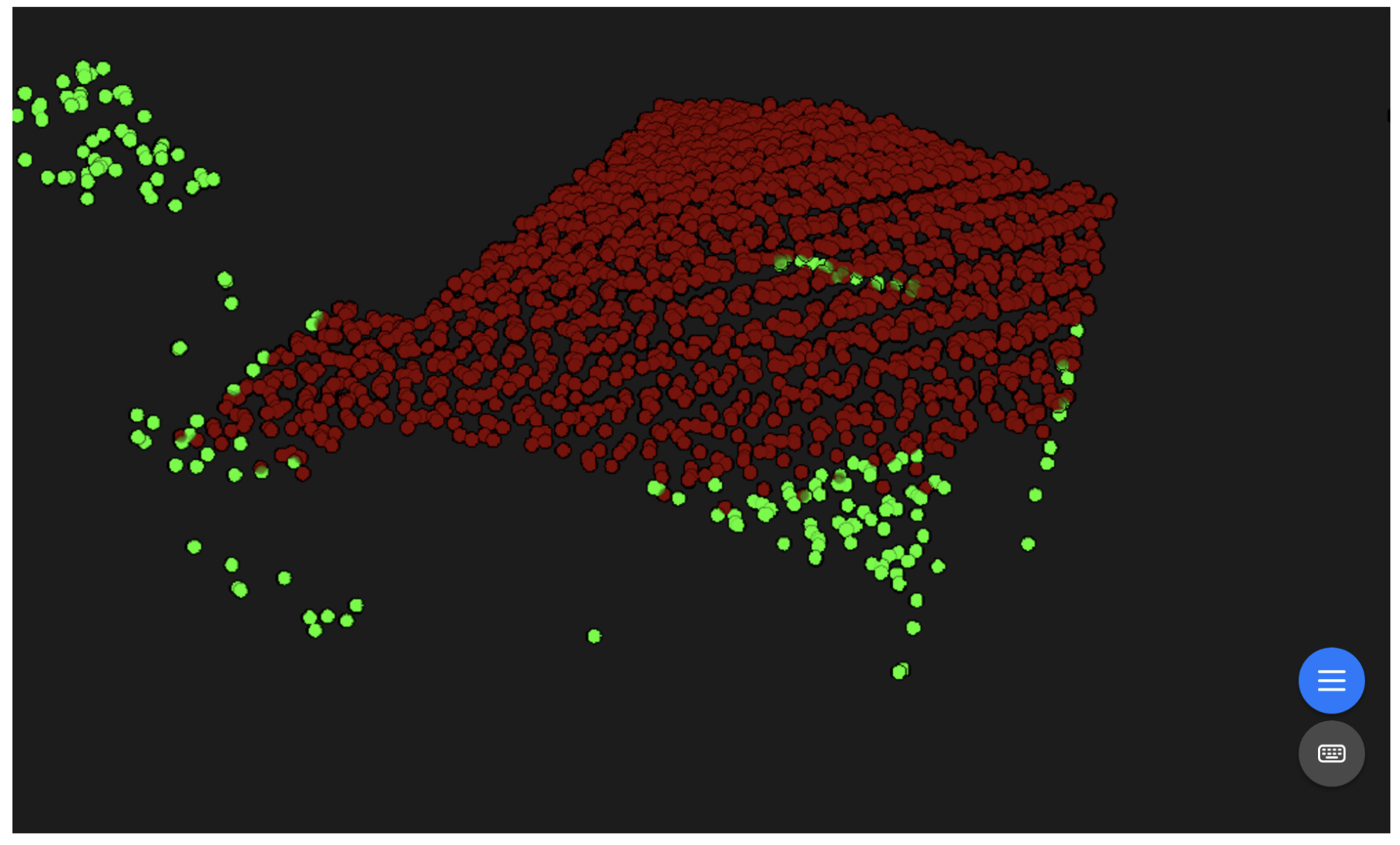I could now extract only the points of the roof and export it as a mesh for further analysis, for instance to calculate the slope of the roof and orientation. But this article is getting long, so I will get to this another time!

The last thing I will show is how to create a 50 meter buffer around the building and use this to look at the surrounding area.

``````buffer = shapely.buffer(shapely.box(*geom.bounds), 50)
buffer
``````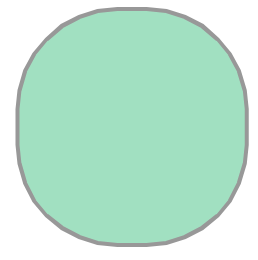As before I read the points from the point cloud array with the buffer I just created:

``````df_surrounds =  gpd.read_file(points_array, engine="pyogrio", bbox=buffer.bounds, use_arrow=True)
df_surrounds["Red"] = 0.0
df_surrounds["Green"] = 0.0
df_surrounds["Blue"] = 0.0

df_surrounds.drop(df_surrounds[(df_surrounds["Classification"] == 7) |
(df_surrounds["Classification"] == 18)|
(df_surrounds["Classification"] == 1)
].index, inplace=True)

msk = df_surrounds.within(buffer)
sp_df = df_surrounds.loc[msk]
``````

Then I fetch the classification codes and color the different classes. And finally use `show.point_cloud()` to create the interactive visualization for the surroundings of the above building:

``````sp_df.Classification.unique()

# ground
sp_df.loc[sp_df["Classification"] == 2, "Red"] = 0.5
sp_df.loc[sp_df["Classification"] == 2, "Green"] = 0.25
# vegetation
sp_df.loc[sp_df["Classification"] == 3, "Green"] = 0.5
sp_df.loc[sp_df["Classification"] == 4, "Green"] = 0.7
sp_df.loc[sp_df["Classification"] == 5, "Green"] = 0.9
# buildings
sp_df.loc[sp_df["Classification"] == 6, "Red"] = 0.5

data = {
"X": sp_df.geometry.x,
"Y": sp_df.geometry.y,
"Z": sp_df.geometry.z,
"Red": sp_df["Red"],
"Green": sp_df["Green"],
"Blue": sp_df["Blue"]
}

show.point_cloud(source="dict",
data=data,
point_size=4,
rgb_max=1.0,
width = 1000,
height = 600)
``````# See you next time!

That's it for TileDB 101: Geometries! You can preview, download or launch the notebook from TileDB Cloud.

Check back soon as there is a lot more to explore with geometry data. Next time I will go into more detail on how the spatial queries work and also will show that they can be used with raster data arrays. TileDB is multi-modal and can handle all geospatial data types through the same APIs.

We'd love to hear what you think of this article. Join our Slack community, or let us know on Twitter and LinkedIn. Look out for future articles in our 101 series on TileDB. Until next time!

Want to see TileDB Cloud in action?Geospatial Data Scientist